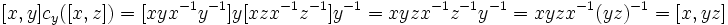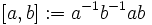# Formula for commutator of element and product of two elements

## Statement

### With the left-action convention

Suppose$G$ is a group and$x,y,z$ are elements of$G$. Then:$\! [x,yz] = [x,y] c_y([x,z])$.

and:$\! [xy,z] = c_x([y,z])[x,z]$

Here$[a,b] = aba^{-1}b^{-1}$ and$c_g(h) = ghg^{-1}$.

### With the right-action convention

Suppose$G$ is a group and$x,y,z$ are elements of$G$. Then:$\! [x,yz] = [x,z][x,y]^z$

and:$\! [xy,z] = [x,z]^y[y,z]$

here$[a,b] = a^{-1}b^{-1}ab$ and$g^h = h^{-1}gh$.

## Proof

### With the left-action convention

Given: A group$G$, elements$x,y,z \in G$

To prove:$\! [x,yz] = [x,y]c_y([x,z])$ and$[xy,z] = c_x([y,z])[x,z]$ where$\! [a,b] := aba^{-1}b^{-1}$ and$c_a(b) = aba^{-1}$.

Proof: We have:$\! [x,y]c_y([x,z]) = [xyx^{-1}y^{-1}]y[xzx^{-1}z^{-1}]y^{-1} = xyzx^{-1}z^{-1}y^{-1} = xyzx^{-1}(yz)^{-1} = [x,yz]$

and:$\! c_x([y,z])[x,z] = x[yzy^{-1}z^{-1}]x^{-1}[xzx^{-1}z^{-1}] = xyzy^{-1}x^{-1}z^{-1} = (xy)z(xy)^{-1}z^{-1} = [xy,z]$

### With the right-action convention

Given: A group$G$, elements$x,y,z \in G$

To prove:$\! [x,yz] = [x,z][x,y]^z$ and$[xy,z] = [x,z]^y[y,z]$ where$[a,b] := a^{-1}b^{-1}ab$ and$a^b = b^{-1}ab$.

Proof: We have:$\! [x,z][x,y]^z = [x^{-1}z^{-1}xz]z^{-1}[x^{-1}y^{-1}xy]z = x^{-1}z^{-1}y^{-1}xyz = x^{-1}(yz)^{-1}x(yz) = [x,yz]$

and:$\! [x,z]^y[y,z] = y^{-1}[x^{-1}z^{-1}xz]y[y^{-1}z^{-1}yz] = y^{-1}x^{-1}z^{-1}xyz = (xy)^{-1}z^{-1}(xy)z = [xy,z]$